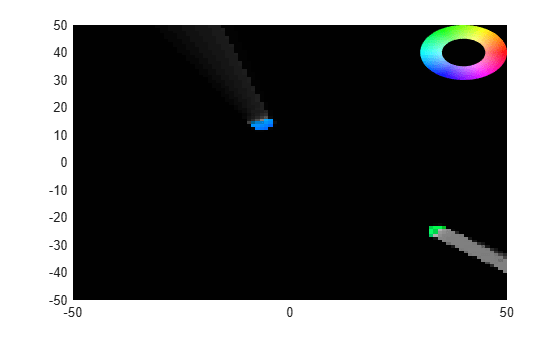dynamicEvidentialGridMap

Dynamic grid map output from trackerGridRFS

Description

The dynamicEvidentialGridMap object represents the dynamic map estimate obtained from the grid-based tracker, trackerGridRFS. You can visualize the dynamic map and obtain the estimated values by using the object functions of dynamicEvidentialGridMap. The dynamicEvidentialGridMap object is a handle object.

Creation

You can generate a dynamicEvidentialGridMap object using a trackerGridRFS object. See the Usage syntax of trackerGridRFS and the Obtain Estimated Values at Grid Level using dynamicEvidentialGridMap example for details.

Properties

expand all

Motion model for tracking, specified as 'constant-velocity', 'constant-acceleration', or 'constant-turn-rate'. The particle state and object state for each motion model are:

MotionModelParticle StateObject State
'constant-velocity'[x; vx; y; vy] [x; vx; y; vy; yaw; L; W]
'constant-acceleration'[x; vx; ax; y; vy; ay][x; vx; ax; y; vy; ay; yaw; L; W]
'constant-turn-rate'[x; vx; y; vy; w][x; vx; y; vy; w; yaw; L; W]

where:

• x — Position of the object in the x direction of the tracking frame (m)

• y — Position of the object in the y direction of the tracking frame (m)

• vx — Velocity of the object in the x direction of the tracking frame (m/s)

• vy — Velocity of the object in the y direction of the tracking frame (m/s)

• ax — Acceleration of the object in the x direction of the tracking frame (m/s2)

• ay — Acceleration of the object in the y direction of the tracking frame (m/s2)

• w — Yaw-rate of the object in the tracking frame (degree/s)

• yaw — Yaw angle of the object in the tracking frame (deg)

• L — Length of the object (m)

• W — Width of the object (m)

Number of state variables corresponding to the motion model specified in the MotionModel property, specified as a positive integer.

Example: 6

x-direction dimension of the grid in the local coordinates, specified as a positive scalar in meters.

y-direction dimension of the grid in the local coordinates, specified as a positive scalar in meters.

Resolution of the grid, specified as a positive scalar. GridResolution represents the number of cells per meter of the grid for both the x- and y-direction of the grid.

Location of the grid origin in the local coordinate frame, specified as a two-element real-valued vector in meters. The grid origin represents the bottom-left corner of the grid map.

Object Functions

 getEvidences Get estimated occupancy and free evidences getOccupancy Get estimated occupancy probabilities getState Get full estimated state and associated uncertainty getVelocity Get estimated velocity and associated uncertainty show Visualize dynamic evidential grid map

Examples

collapse all

Create a tracking scenario.

rng(2021);% For reproducible results
scene = trackingScenario('UpdateRate',5,'StopTime',5);

Add a platform. Mount a lidar sensor on the platform.

plat = platform(scene);
lidar = monostaticLidarSensor(1,'DetectionCoordinates','Body');

Add two targets and define their position, velocity, orientation, dimension, and meshes.

for i = 1:2
target = platform(scene);
x = 50*(2*rand-1);
y = 50*(2*rand-1);
vx = 5*(2*rand-1);
vy = 5*(2*rand-1);
target.Trajectory.Position = [x y 0];
target.Trajectory.Velocity = [vx vy 0];
target.Trajectory.Orientation = quaternion([atan2d(vy,vx),0,0],'eulerd','ZYX','frame');
target.Mesh = extendedObjectMesh('sphere');
target.Dimensions = struct('Length',4, ...
'Width',4, ...
'Height',2, ...
'OriginOffset',[0 0 0]);
end

Define the configuration of the sensor.

config = trackingSensorConfiguration(1, ...
'SensorLimits',[-180 180;0 100], ...
'SensorTransformParameters',struct, ...
'IsValidTime',true);

Create a grid-based tracker.

tracker = trackerGridRFS('SensorConfigurations',config, ...
'AssignmentThreshold',5, ...
'MinNumCellsPerCluster',4, ...
'ClusteringThreshold',3);

Advance scenario and run the tracker based on the lidar data.

% Current time
time = scene.SimulationTime;

% Generate point cloud
tgtMeshes = targetMeshes(plat);
[ptCloud, config] = lidar(tgtMeshes, time);

% Format the data for the tracker
sensorData = struct('Time',time, ...
'SensorIndex',1, ...
'Measurement',ptCloud', ...
'MeasurementParameters',struct ...
);

% Call tracker using sensorData to obtain the map in addition
% to tracks
[tracks, ~, ~, map] = tracker(sensorData,time);

% Obtain the estimated occupancy probability of each cell
P_occ = getOccupancy(map);

% Obtain the estimated evidences for each cell
[m_occ, m_free] = getEvidences(map);

% Obtain the estimated velocity for each cell
[v, Pv] = getVelocity(map);

% Obtain the estimated state for each cell
[x, P] = getState(map);

% Show the map
show(map,'InvertColors',true)
end